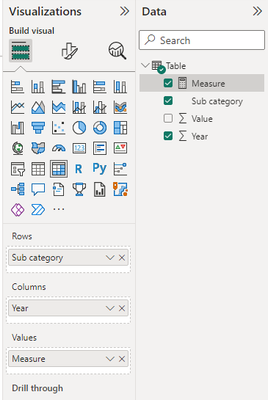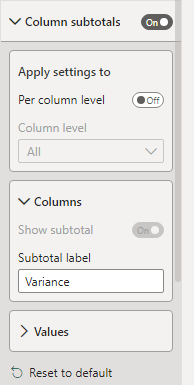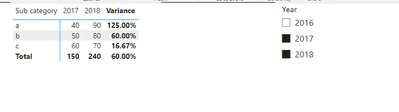cancel
Showing results for
Did you mean:Regular Visitor

## Variance calculation in Table or Matrix

Hi everyone

I'm doing a sales comparison report in which the end user can pick any 2 dates in a slicer (2016 and 2021 in below example).To show the results, I'm trying to achieve this visual made with excel :In PBI I used a table and a matrix to visualize the results but i can't replicate the excel table above.

1/ TABLE

I went first for a table for which I created 3 measures :

a) SalesTotal_YearOldestCALCULATE([SalesTotal]FILTER(CALENDRIERCALENDRIER[Year]=MIN(CALENDRIER[Year] ))) ----- to show 2016 results

b) SalesTotal_YearMostRecent = CALCULATE([SalesTotal], FILTER(CALENDRIER, CALENDRIER[Year]=MAX(CALENDRIER[Year] ))) ----- to show 2021 results

c) Variance = [SalesTotal_YearMostRecent] - [SalesTotal_YearOldest] / [SalesTotal_YearOldest]

Here's the visual :This table is ok but i can't find a way to have dynamic column headers : YEAR(oldest) would be 2016 and YEAR(most recent) would be 2021 (based on the years picked in the slicer).

I've read many posts on dynamic column headers but it seems it's not possible for tables (unless you have an idea on how to do it ?).

So i opted for a matrix.

2/ MATRIX

Here's the visual without the Variance measure :Problem is i can't use the Variance measure in that matrix. The measure won't stay in the "Colonnes" field when i try to drag and drop the measure in. And when i put it in the "Valeurs" field, here's what it does :Can anyone help me out reproducing the screenshot excel table please ?

Here's the data model if it can be of any help :I hope my explanations are clear and detailed enough.

Thank you 🙏

1 ACCEPTED SOLUTIONCommunity Support

Hi @AnthNC ,

Based on your description, I have created a simple example:``````Measure =
var _a = CALCULATE(SUM('Table'[Value]),FILTER('Table',[Year]=MIN('Table'[Year])))
var _b = CALCULATE(SUM('Table'[Value]),FILTER('Table',[Year]=MAX('Table'[Year])))
return
IF(ISINSCOPE('Table'[Year]),SUM('Table'[Value]),FORMAT(DIVIDE(_b-_a,_a),"Percent"))``````Change the name of  column subtotal:Final output:Best Regards,

Jianbo Li

If this post helps, then please consider Accept it as the solution to help the other members find it more quickly.

2 REPLIES 2Community Support

Hi @AnthNC ,

Based on your description, I have created a simple example:``````Measure =
var _a = CALCULATE(SUM('Table'[Value]),FILTER('Table',[Year]=MIN('Table'[Year])))
var _b = CALCULATE(SUM('Table'[Value]),FILTER('Table',[Year]=MAX('Table'[Year])))
return
IF(ISINSCOPE('Table'[Year]),SUM('Table'[Value]),FORMAT(DIVIDE(_b-_a,_a),"Percent"))``````Change the name of  column subtotal:Final output:Best Regards,

Jianbo Li

If this post helps, then please consider Accept it as the solution to help the other members find it more quickly.Regular Visitor

Many thanks Jianbo Li !

I'm finding out about how to use variables in measures. Thanks again